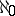# Cardinality

(diff) ← Older revision | Latest revision (diff) | Newer revision → (diff)
cardinal number, of a setThat property ofwhich is inherent in any setequivalent to. Here two sets are called equivalent (or equipotent or of the same cardinality) if it is possible to construct a one-to-one correspondence between them. Thus, "defining by abstraction" , one may say that cardinality is that which is common to all equivalent sets. Since what is common to all finite sets equivalent to each other is the quantity, or number, of their elements, in its application to infinite sets the idea of cardinality is an analogue of the idea of quantity. The cardinality is a fundamental idea in set theory, due to G. Cantor. A set that is equivalent to the set of all natural numbers is called countable. The corresponding cardinality is denoted by(aleph null). The cardinality of sets equivalent to the set of real numbers is called the cardinality of the continuum and is denoted byor. For example, the set of all algebraic numbers has countable cardinality, and the set of all closed subsets of-dimensional Euclidean space has the cardinality of the continuum. The Cantor–Bernshtein theorem: If for two sets, each is equivalent to a subset of the other, then the two sets are equivalent. In this case it is said that these sets have the same cardinality. If a setis equivalent to a subset of a set, whereasis not equivalent to any subset of, then it is said that the cardinality ofis greater than that of. Cantor's theorem: The cardinality of the set of all subsets of a non-empty setis greater than the cardinality of. This theorem allows one to construct a hierarchy of cardinalities (see Cardinal number).